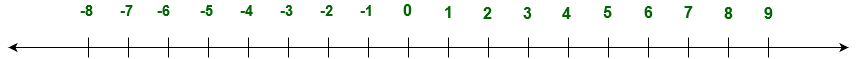# GRE Arithmetic | Integers

There is a different type of numbers: natural number, real number, whole number, integers etc. Integers are the numbers -1, -2, -3, -4, 1, 2, 5, 9, -10. Integers are either negative or positive (0 is the exception which is neither negative nor positive integer).

Let’s understand it by number line:Types of integers:

• Positve integer (e.g. 5, 8, 12, 29 etc).
• Negative integer (e.g. -5, -8, -12, -29 etc).
• Even integers (e.g. 4, 8, 12, 28, -28, -12, -8, -4 etc).
• Odd integers (e.g. 5, 9, 13, 29, -33. -31, -49 etc).
• Neither positive nor negative integer is 0.
• Prime number is an integer which is divisible by only two positive integer (1 and the integer itself, e.g. 2, 3, 5, 7, 11, 29 etc).
• Coprime number is pair of two positive integer which have 1 as a only common positive divisor.

What is least common multiple and greatest common divisor ?
Least common divisor (LCM): It is the least common multiple of two positive integer.

```Example:
6 is the LCM of 2 and 3 ```

Greatest common divisor (GCD): It is the greatest common factor (GCF) of two positive integer.

```Example:
GCD of 15 and 30 is 15 ```

Different type of operation can be performed on integers:

1. Addition of two integer will be an integer (negative or positive).
2. Subtraction of two integer will be an integer (negative or positive).
3. Product of two integer will be an integer (negative or positive).
4. Division of two integer can be an integer or decimal (negative or positive).
5. Square of an integer (negative or positive) will be a positive integer.
6. Root of a positive integer can be an integer or decimal.
```odd + odd = even
odd - odd = even
even + even = even
even - even = even
odd + even = odd
odd - even = even
Even - odd = odd
odd * odd = odd
even * even = even
odd * even = even ```

For example:

```3 + 3 = 6
(-3) + (-3) = -6
3 - 1 = 2
(-3) - (-1) = -2
4 + 4 = 8
(-4) + (-4) = -8
4 - 2 = 2
(-4) - (-2) = (-2)
3 + 4 = 7
(-3) + (-4) = (-7)
5 - 2 = 3
(-5) - (-2) = (-3)
5 - 3 = 2
(-5) - (-3) = (-2)
3 * 3 = 9
(-3) * (-3) = 9
4 * 4 = 16
(-4) * (-4) = 16
3 * 4 = 12
(-3) * (-4) = 12
√4 = 2
√2 = 1.41421...
6 / 2 = 3
(-6) / (-2) = 3
(-6) / 2 = -3
6 / (-2) = -3
5 / 2 = 2.5
(-5) / (-2) = 2.5
5 / (-2) = -2.5
(-5) / 2 = -2.5
```

My Personal Notes arrow_drop_upCheck out this Author's contributed articles.

If you like GeeksforGeeks and would like to contribute, you can also write an article using contribute.geeksforgeeks.org or mail your article to contribute@geeksforgeeks.org. See your article appearing on the GeeksforGeeks main page and help other Geeks.

Please Improve this article if you find anything incorrect by clicking on the "Improve Article" button below.

Article Tags :

Be the First to upvote.

Please write to us at contribute@geeksforgeeks.org to report any issue with the above content.